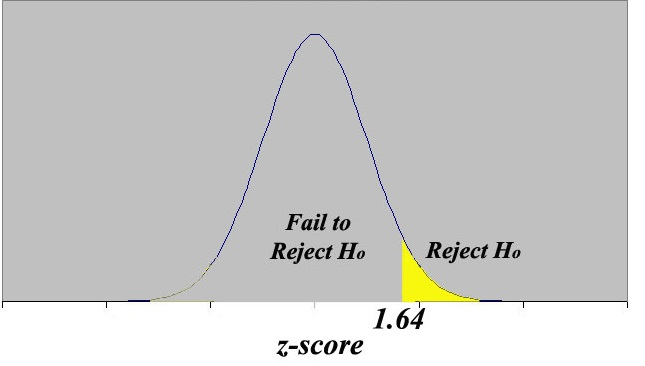# AP Statistics : How to do tests of significance for a difference between two means

## Example Questions

### Example Question #5 : Mean And Linear Regression

Suppose you conduct a paired-test to assess whether two group means significantly differ and find a-score of 1.645. At what alpha level would this cause you to reject the null?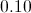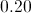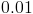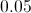Explanation:

The critical value for a-test with alpha set to 0.10 is 1.645.

### Example Question #6 : Mean And Linear Regression

Bob wants to statistically determine if the mean height of middle school boys is greater than the mean height of middle school girls. He wants to use a significance level of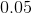What must be true for him to reject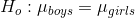.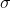is known.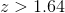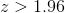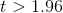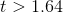Explanation:

Step 1: We need to use a 2-sample z test because there are 2 samples, boys and girls. The population standard deviation,, is known, so we can assume a standard distribution for each sample.

Step 2: This is a one-sided z test because the questions asks if the mean height of boys is greater than the mean height of girls.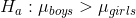Step 3: significance level, or alpha, is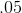. This means we need a p-value less thanin order to reject the null hypothesis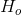. A z-score greater than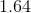would ensure a p-value less than.